﻿ 大别山地形对降水的增幅作用研究 Study of Ta-Pieh Mountains Terrain on the Precipitation Enhancement

Climate Change Research Letters
Vol.07 No.02(2018), Article ID:23989,8 pages
10.12677/CCRL.2018.72008

Study of Ta-Pieh Mountains Terrain on the Precipitation Enhancement

Ni Liu1,2, Shanshan Hu3, Yiwu Xu1

1Huangshan Meteorological Office of Anhui Province, Huangshan Anhui

2Anhui Meteorological Observatory, Hefei Anhui

3Chuzhou Meteorological Observatory of Anhui Province, Chuzhou Anhui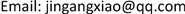Received: Feb. 18th, 2018; accepted: Mar. 2nd, 2018; published: Mar. 9th, 2018ABSTRACT

In order to explore the effect of the Ta-pieh mountain range on the increase of precipitation, by using conventional LAPS and DEM data in the Chinese Academy of Sciences, this paper describes the climate distribution of precipitation and extreme precipitation in the Ta-pieh Mountains, analyzes the effect of Ta-pieh Mountains terrain on the precipitation enhancement during June 29 to 30 June of 2009, uses the WRF model to test the terrain sensitivity, and finally calculates the vapor convergence rainfall and vapor vertical transport increment. According to the climate scale, the results show that the precipitation is closely related to the Ta-pieh Mountains terrain, the distribution of extreme rainfall has obvious local characteristics, high altitude areas and the southwest slope are more prone to extreme rainfall. During the precipitation process, the precipitation is positively correlated with the altitude, which rises rapidly with the increase of height on the windward slope. WRF sensitivity test shows that the intensity of the heavy rainfall distribution is obviously affected by the terrain, and the enhancement of the rainfall is large. From the results of the vapor convergence rainfall and vapor vertical transport increment, the enhancements of rainfall in high altitude areas and the windward slope are less linked to precipitation background, which mainly derives from vapor vertical transport increment caused by the uplift of the mountain.

Keywords:Ta-Pieh Mountains, Extreme Rainfall, Terrain, Enhancement

1安徽省黄山气象管理处，安徽 黄山

2安徽省气象台，安徽 合肥

3安徽省滁州市气象台，安徽 滁州Copyright © 2018 by authors and Hans Publishers Inc.

This work is licensed under the Creative Commons Attribution International License (CC BY).

http://creativecommons.org/licenses/by/4.0/1. 引言

2. 资料与方法

2.1. 资料

2.2. 方法

$I=-\frac{1}{g}{\int }_{0}^{{p}_{0}}\frac{\text{d}q}{\text{d}t}\text{d}p$ (1)

$\frac{\text{d}q}{\text{d}t}=\frac{\partial q}{\partial t}+V\cdot \nabla q+\omega \frac{\partial q}{\partial p}$ (2)

$I=-\frac{1}{g}{\int }_{0}^{{p}_{0}}\frac{\partial q}{\partial t}\text{d}p-\frac{1}{g}{\int }_{0}^{{p}_{0}}\nabla \cdot Vq\text{d}p-\frac{1}{g}{\int }_{0}^{{p}_{0}}\omega q$ (3)

1) 水汽通量散度整层积分计算

$I\approx -\frac{1}{g}{\int }_{0}^{{p}_{0}}\nabla \cdot Vq\text{d}p$ (4)

2) 水汽垂直输送增量计算

3) 增幅系数方法估算

$R\left(x,y,t\right)=k\left(x,y\right)\cdot f\left(x,y,t\right)$

$f\left(x,y,t\right)$ 为没有地形影响时扰动经过测站的降水，简称扰动降水； $k\left(x,y\right)$ 是在某地的增幅因子，设扰动系数为

$h\left(x,y,t\right)=R\left(x,y,t\right)/\stackrel{¯}{R}\left(x,y,t\right)$

$h\left(x,y,t\right)=R\left(x,y,t\right)/\stackrel{¯}{R}\left(x,y,t\right)$

$R\left(x,y,t\right)=k\left(x,y\right)\cdot f\left(x-vt\right)$

${x}_{2}-{x}_{1}=v\left({t}_{2}-{t}_{1}\right)$

$f\left({x}_{2}-v{t}_{2}\right)=f\left({x}_{1}-v{t}_{1}\right)$

$\frac{R\left({x}_{1},{y}_{1},{t}_{1}\right)}{R\left({x}_{2},{y}_{2},{t}_{2}\right)}=\frac{k\left({x}_{1},{y}_{1}\right)}{k\left({x}_{2},{y}_{2}\right)}$

$dR=R\left(x,y,t\right)-\stackrel{¯}{R}$

$k=dR/\stackrel{¯}{R}$

3. 大别山降水和极端强降水的气候分布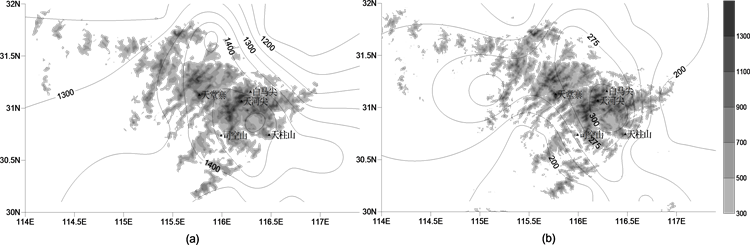Figure 1. (a) Annual average precipitation in the Ta-pieh Mountains region (1981~2010), (b) 24 hours rainfall extreme value distribution (unit: mm, shadow part indicates altitude)

4. 过程强降水增幅分析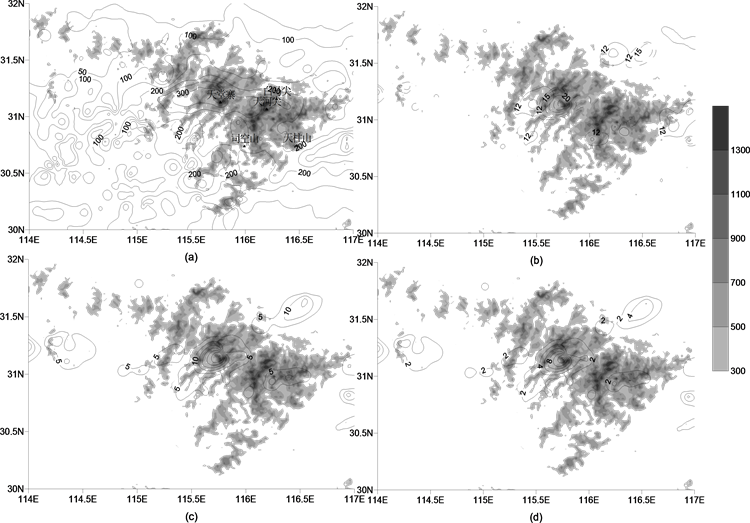Figure 2. (a) Space distribution of the 6.29 heavy rainfall in the Ta-pieh Mountains (unit: mm), (b) Contrast of rainfall center between 20:00 (solid line) and 21:00 (dashed line) in June 29, 2009 (unit: mm), (c) Rainfall increment at 21:00 calculating by the method of increase coefficient (unit: mm), (d) Distribution of coefficient K value at 21:00, the shading indicates the altitude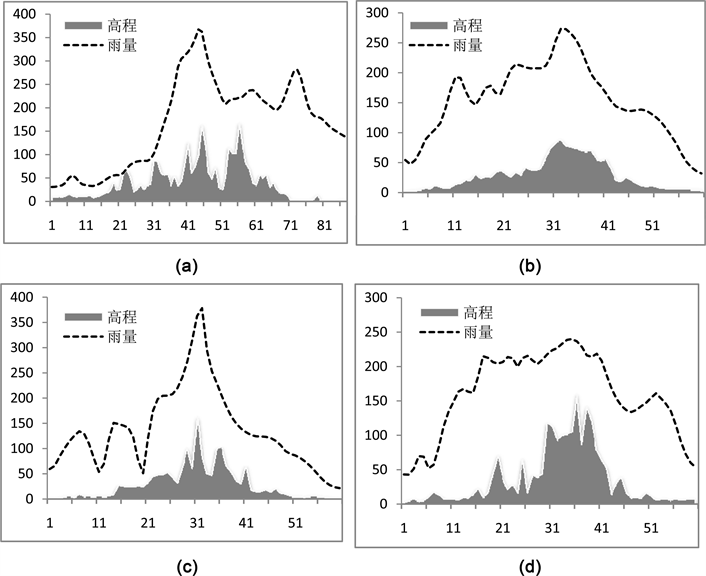Figure 3. (a) Vertical profile of the precipitation along the Ta-pieh Mountains (Heaven village to Sikong mountain), (b) Vertical profile of mean precipitation on upwind slope, (c) Vertical profile of precipitation on upwind slope along the top of Heaven village, (d) Vertical profile of precipitation on upwind slope along the top of Sikong mountain (unit: mm)

5. 地形对强降水的增幅计算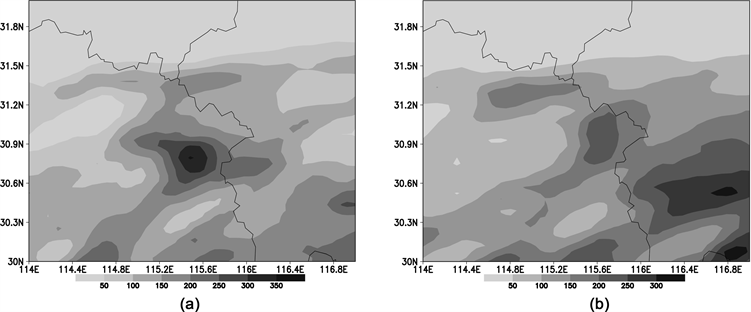Figure 4. (a) WRF rainfall distribution with topographic effects, (b) Same as (a), but no effect of topography (unit: mm)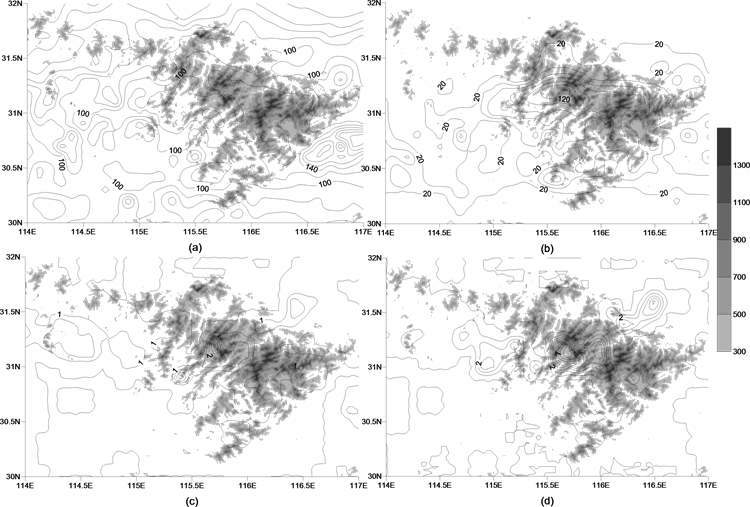Figure 5. (a) Rainfall distribution of water vapor calculating by convergence, (b) Rainfall distribution of water vapor calculating by the vertical transfer increment, (c) Rainfall difference of water vapor calculating by convergence between 21:00 and 20:00, (d) Rainfall difference of water vapor calculating by the vertical transfer increment between 21:00 and 20:00 (unit: mm)

6. 结论

 林必元, 张维恒. 地形对降水影响的研究[M]. 北京: 气象出版社, 2001.

 慕建利, 李泽春, 李耀辉, 等. 高原东侧特大暴雨过程中秦岭山脉的作用[J]. 高原气象, 2009, 28(6): 1282-1290.

 廖移山, 冯新, 石燕, 等. 2008年“7.22”襄樊特大暴雨的天气学机理分析及地形的影响[J]. 气象学报, 2011, 69(6): 945-955.

 廖菲, 洪延超, 郑国光. 地形对降水的影响研究概述[J]. 气象科技, 2007, 35(3): 305-316.

 李子良, 地形降水试验和背风坡回流降水机制[J]. 气象, 2006, 32(5): 10-15.

 林之光. 地形降水气候学[M]. 北京: 科学出版社, 1995: 91.

 丁仁海, 王龙学. 九华山暴雨地形增幅作用的观测分析[J]. 暴雨灾害, 2009, 28(4): 377-381.

 张美根, 韩志伟, 雷孝恩. 地形性中尺度环流的数值模拟及验证[J]. 大气科学, 2000, 24(1): 122-130.

 崔春光, 李红莉, 彭菊香, 等. LAPS资料在一次鄂东初夏暴雨分析中的应用[J]. 暴雨灾害, 2008, 27(4): 307-312.

 刘裕禄, 黄勇. 黄山山脉地形对暴雨降水增幅条件研究[J]. 高原气象, 2013, 32(2): 608-618.

 董美莹, 陈联寿, 程正泉. 地形影响热带气旋“泰利”降水增幅的数值研究[J]. 高原气旋, 2011, 30(3): 700-710.

 朱乾根, 林景瑞, 寿绍文, 等著. 天气学原理和方法[M]. 北京: 气象出版社, 2002: 636-643.

 黄玲琳. 地形对降水增幅作用的机理探讨[J]. 浙江气象科技, 1993, 14(2): 11-14.

 徐昕, 王其伟, 王元. 迎风坡降水对中国东南地区降水贡献的估测[J]. 南京大学学报(自然科学版), 2010, 46(6): 56-59.